Contemporary Mathematics

# 12.4Navigating Graphs

Contemporary Mathematics12.4 Navigating Graphs

Figure 12.77 Visitors navigate a garden maze. (credit: “Longleat Maze” by Niki Odolphie/Wikimedia, CC BY 2.0)

### Learning Objectives

After completing this section, you should be able to:

1. Describe and identify walks, trails, paths, and circuits.
2. Solve application problems using walks, trails, paths, and circuits.
3. Identify the chromatic number of a graph.
4. Describe the Four-Color Problem.
5. Solve applications using graph colorings.

Now that we know the basic parts of graphs and we can distinguish one graph from another, it is time to really put our graphs to work for us. Many applications of graph theory involve navigating through a graph very much like you would navigate through a maze. Imagine that you are at the entrance to a maze. Your goal is to get from one point to another as efficiently as possible. Maybe there are treasures hidden along the way that make straying from the shortest path worthwhile, or maybe you just need to get to the end fast. Either way, you definitely want to avoid any wrong turns that would cause unnecessary backtracking. Luckily, graph theory is here to help!

### Walks

Suppose Figure 12.78 is a maze you want to solve. You want to get from the start to the end.

You can approach this task any way you want. The only rule is that you can’t climb over the wall. To put this in the context of graph theory, let’s imagine that at every intersection and every turn, there is a vertex. The edges that join the vertices must stay within the walls. The graph within the maze would look like Figure 12.79.

Figure 12.79 The Graph in Your Maze

One approach to solving a maze is to just start walking. It is not the most efficient approach. You might cross through the same intersection twice. You might backtrack a bit. It’s okay. We are just out for a walk. It might look something like the black sequence of vertices and edges in Figure 12.80.

Figure 12.80 The Walk Through the Graph in Your Maze

This type of sequence of adjacent vertices and edges is actually called a walk (or directed walk) in graph theory too!

A walk can be identified by naming the sequence of its vertices (or by naming the sequence of its edges if those are labeled). Let’s take the graph out of the context of the maze and give each vertex a name and each edge of the walk a direction as in Figure 12.81.

Figure 12.81 The Graph without Your Maze

The name of this walk from p to r is pqonijcdcjksr. When a particular edge on our graph was traveled in both directions, it had arrows in both directions and the letters of vertices that were visited more than once had to be repeated in the name of the walk.

### Checkpoint

Not every list of vertices or list of edges makes a path. The order must take into account the way in which the edges and vertices are connected. The list must be a sequence, which means they are in order. No edges or vertices can be skipped and you cannot go off the graph.

Figure 12.82 A Walk or Not a Walk?

The highlighted edges Graph Y in Figure 12.82 represent a walk between f and b. The highlighted edges in Graph X do not represent a walk between f and b, because there is a turn at a point that is not a vertex. This is like climbing over a wall when you are walking through a maze. Another way of saying this is that bdf is not a walk, because there is no edge between b and d.

### Checkpoint

A point on a graph where two edges cross is not a vertex.

### Example 12.19

#### Naming a Walk Through A House

Figure 12.83 shows the floor plan of a house. Use the floor plan to answer each question.

Figure 12.83 Floor Plan of a House
1. Draw a graph to represent the floor plan in which each vertex represents a different room (or hallway) and edges represent doorways between rooms.
2. Name a walk through the house that begins in the living room, ends in the garage and visits each room (or hallway) at least once.

1.
Recall from the Graph Basics section that a graph in which there are multiple edges between the same pair of vertices is called a multigraph. The figure shows a map of the four bridges that link Staten Island to Brooklyn and New Jersey and a multigraph representing the map. In the multigraph, the edges are labeled instead of the vertices. The edges represent the bridges, G (Goethals Bridge), B (Bayonne Bridge), C (Outerbridge Crossing), and V (Verrazzano-Narrows Bridge). The vertices represent New Jersey, Staten Island, and Brooklyn.
Figure 12.87 Map and Multigraph of Staten Island Bridges
A path can be named by a sequences of edges instead of a sequence of vertices. Determine which of the following sequence of edges is a walk.
1. BVCGC
2. VCBGC
3. CVGBB
4. GVBVC

### Paths and Trails

A walk is the most basic way of navigating a graph because it has no restrictions except staying on the graph. When there are restrictions on which vertices or edges we can visit, we will call the walk by a different name. For example, if we want to find a walk that avoids travelling the same edge twice, we will say we want to find a trail (or directed trail). If we want to find a walk that avoids visiting the same vertex twice, we will say, we want to find a path (or directed path).

Walks, trails, and paths are all related.

1. All paths are trails, but trails that visit the same vertex twice are not paths.
2. All trails are walks, but walks in which an edge is visited twice would not be trails.

We can visualize the relationship as in Figure 12.88.

Figure 12.88 Walks, Trails, and Paths

Let’s practice identifying walks, trails, and paths using the graphs in Figure 12.89.

Figure 12.89 Graphs A and K

### Example 12.20

#### Identifying Walks, Paths, and Trails

Consider each sequence of vertices from Graph A in Figure 12.89. Determine if it is only a walk, both a walk and a path, both a walk and a trail, all three, or none of these.

1. bcdef
2. cbdbe
3. cfedbc
4. befcbd

Consider each sequence of vertices from Graph K in Figure 12.106. Determine if it is only a walk, both a walk and a path, both a walk and a trail, all three, or none of these.
1.
nqop
2.
pnqonm
3.
mnopq

### Circuits

In many applications of graph theory, such as creating efficient delivery routes, beginning and ending at the same location is a requirement. When a walk, path, or trail end at the same location or vertex they began, we call it closed. Otherwise, we call it open (does not begin and end at the same location or vertex). Some examples of closed walks, closed trails, and closed paths are given in in the following table.

DESCRIPTION EXAMPLE CHARACTERISTICS
A closed walk is a walk that begins and ends at the same vertex.

dfbcfd

Alternating sequence of vertices and edges

Begins and ends at the same vertex

A closed trail is a trail that begins and ends at the same vertex. It is commonly called a circuit.

dfbcfed

No repeated edges

Begins and ends at the same vertex

A closed path is a path that begins and ends at the same vertex. It is also referred to as a directed cycle because it travels through a cyclic subgraph.

dfbcd

No repeated edges or vertices

Begins and ends at the same vertex

Table 12.4 Closed Walks, Trails, and Paths

Since walks, trails, and paths are all related, closed walks, circuits, and directed cycles are related too.

1. All circuits are closed walks, but closed walks that visit the same edge twice are not circuits.
2. All directed cycles are circuits, but circuits in which a vertex is visited twice are not directed cycles.

We can visualize the relationship as in Figure 12.94.

Figure 12.94 Closed Walks, Circuits, and Directed Cycles

The same circuit can be named using any of its vertices as a starting point. For example, the circuit dfbcd can also be referred to in the following ways.

abcda is the same as

${ b→c→d→a→bc→d→a→b→cd→a→b→c→d{ b→c→d→a→bc→d→a→b→cd→a→b→c→d$

Let’s practice working with closed walks, circuits (closed trails), and directed cycles (closed paths). In the graph in Figure 12.95, the vertices are major central and south Florida airports. The edges are direct flights between them.

Figure 12.95 Major Central and South Florida Airports

### Example 12.21

#### Determining a Closed Walk, Circuit, or Directed Cycle

Suppose that you need to travel by air from Miami (MIA) to Orlando (MCO) and you were restricted to flights represented on the graph. For the trip to Orlando, you decide to purchase tickets with a layover in Key West (EYW) as shown in Figure 12.96, but you still have to decide on the return trip. Determine if your roundtrip itinerary is a closed walk, a circuit, and/or a directed cycle, based on the return trip described in each part.

Figure 12.96 MIA to EYW to MCO
1. You returned to Miami (MIA) by reversing your route.
2. Your direct flight back left Orlando (MCO) but was diverted to Fort Lauderdale (FLL)! From there you flew to Tampa (TPA) before returning to Miami (MIA).

Suppose that you want to travel from Palm Beach (PBI) to any other city listed in Figure 12.113 and then return to Palm Beach.
1.
Why is it impossible to find an itinerary that is a circuit?
2.
How is this related to the degree of the vertex PBI?

### Graph Colorings

In this section so far, we have looked at how to navigate graphs by proceeding from one vertex to another in a sequence that does not skip any vertices, but in some applications we may want to skip vertices. Remember the camp Olympics at Camp Woebegone in Comparing Graphs? You were planning a camp Olympics with four events. The campers signed up for the events. You drew a graph to help you visualize which events have campers in common. The vertices of Graph E in Figure 12.97 represent the events and adjacent vertices indicate that there are campers who are participating in both.

Figure 12.97 Graphs of Camp Olympics

In this case, we do NOT want events represented by two adjacent vertices to occur in the same timeslot, because that would prevent the campers who wanted to participate in both from doing so. We can use the graph in Figure 12.97 to count the timeslots we need so there are no conflicts. Let’s assign each timeslot a different color. We could categorize events that happen at 1 pm as Red; 2 pm, Purple; 3 pm, Blue; and 4 pm, Green. Then assign different colors to any pair of adjacent vertices to ensure that the events they represent do not end up in the same timeslot. Figure 12.98 shows several of the ways to do this while obeying the rule that no pair of adjacent vertices can be the same color.

Figure 12.98 Graph Colorings

In Figure 12.98, the graphs with vertices colored so that no adjacent vertices are the same color are called graph colorings. Notice that Graph 3 has the fewest colors, which means it shows us how to have the fewest number of timeslots. The events marked in red, a and d, can be held at the same time because they are not adjacent and do not have conflicts. Also, the events marked in purple, b and c, can be held at the same time. We would not need green or blue timeslots at all!

A graph that uses $nn$ colors is called an $nn$-coloring. The smallest number of colors needed to color a particular graph is called its chromatic number.

Graph colorings can be used in many applications like the scheduling scenario at camp Woebegone. Let's look at how they work in more detail. Figure 12.99 shows two different colorings of a particular graph. Coloring A is called a four-coloring, because it uses four colors, red (R), green (G), blue (B), and purple (P). Coloring B is called a three-coloring because it uses three. The colors allow us to visually subdivide the graphs into groups. The only rule is that adjacent vertices are different colors so that they are in different groups.

Figure 12.99 Two Colorings of the Same Graph

It turns out that a three-coloring is the best we can do with the graph in Figure 12.99. No matter how many different patterns you try with only two colors, you will never find one in which the adjacent vertices are always different colors. In other words, the graph has a chromatic number of three. For large graphs, computer assistance is usually required to find the chromatic numbers. There is no formula for finding the chromatic number of a graph, but there are some facts that are helpful in Table 12.5.

Fact Example
Recall that planar graphs are untangled; that is, they can be drawn on a flat surface so that no two edges are crossing. If a graph is planar, it can be colored with four colors or possibly fewer.
Each vertex in a complete graph is adjacent to every other vertex forcing us to color them all different colors.The chromatic number of a complete graph is the same as the number of vertices.
If a graph has a clique, a complete subgraph, then each vertex in the clique must have a different color and the vertices outside the clique may or may not have even more colors. The chromatic number of a graph is at least the number of vertices in its biggest clique.
Table 12.5 Facts about Graph Colorings

### Creating Colorings to Solve Problems

Let’s see how these facts can help us color the graph in Figure 12.100.

• Since the graph is planar, the chromatic number is no more than four.
• The graph is not complete, but it has complete subgraphs of three vertices. In other words, it has triangles like the one shown with blue vertices in Figure 12.100. This means that the chromatic number is at least three.
Figure 12.100 A Graph with Triangles

We know we can color this graph in three or four colors. It is usually best to start by coloring the vertex of highest degree as shown in Figure 12.101. In this case, we used red (R). The color is not important.

Figure 12.101 Color Highest Degree Vertex First

We want to color as many of the vertices the same color as possible; so, we look at all the vertices that are not adjacent to the red vertex and begin to color them, red starting with the one among them of highest degree. Since the only vertices that are not adjacent are both degree 2, choose either one and color it red as shown in Figure 12.102.

Figure 12.102 Color More Red from Highest to Lowest Degree

Now, there is only one vertex left that is not adjacent to a red; so, color it red. Of the remaining vertices, the highest degree is four; so, color one of the vertices of degree four in a different color. These two steps are shown in Figure 12.103.

Figure 12.103 Complete the Reds and Start Another Color

Repeat the same procedure. There are three remaining vertices that are not adjacent to a blue. Color as many blue as possible, with priority going to vertices of higher degree as shown in Figure 12.104.

Figure 12.104 Repeat Procedure for Color Blue

All the remaining vertices are adjacent to blue. So, it is time to repeat the procedure with another color as shown in Figure 12.105.

Figure 12.105 Repeat Procedure for Color Purple

All the vertices are now colored with a three-coloring so we know the chromatic number is at most three, but we knew the chromatic number was at least three because the graph has a triangle. So, we are now certain it is exactly three.

### Video

Suppose the vertices of the graph in Figure 12.106 represented the nine events in the Camp Woebegone Olympics, and edges join any events that have campers in common, but nonadjacent vertices do not.

Figure 12.106 Coloring for Nine Event Camp Woebegone Olympics

Any vertices of the same color are nonadjacent; so, they have no conflicts. Since this graph is a three-coloring, all nine events could be scheduled in just three timeslots!

### Example 12.22

#### Understanding Chromatic Numbers

In Example 12.17, we discussed a high school, which holds end-of-course exams in (E3) English 3, (E4) English 4, (M) Advanced Math, (C) Calculus, (W) World History, (U) U.S. History, (B) Biology, and (P) Physics. We were given a list of courses that had no students in common. We used that information to find the graph in Figure 12.71, which shows edges between exams with students in common. Use the graph we found in Example 12.17 to answer each question.

Figure 12.107 Graph of Exams with Students in Common
1. The graph contains a clique of size 4 formed by the vertices P, E3, C, and U. What does this tell you about the chromatic number?
2. The graph is not planar, meaning that you cannot untangle it. What does this tell you about the chromatic number?
3. Create a coloring by coloring vertex of highest degree first, coloring as many other vertices as possible each color from highest to lowest degree, then repeating this process for the remaining vertices.
4. Do you know what the minimum number of timeslots is? If so, what is it and how do you know? If not, what are the possibilities?

### Checkpoint

The procedure used in Example 22 to color the graph is not guaranteed to result in a graph that has the minimum number of colors possible, but it is usually results in a coloring that is close to the chromatic number.

The given figure shows the same graph colored in several ways. The largest clique of the graph has three vertices. Use this information to answer each question.
Figure 12.108 Possible Colorings of the Same Graph
1.
2.
What is the lowest possible chromatic number of this graph based on the size of any cliques?
3.
What are the possible chromatic numbers?
4.
Which graph or graphs meet the definition of a valid graph coloring?
5.
Draw an n-coloring for the graph where n is the answer to Exercise 1.

### The Four Color Problem

Figure 12.109 Using only four colors, no two adjacent regions have the same color.

The idea of coloring graphs to solve problems was inspired by one of the most famous problems in mathematics, the “four color problem.” The idea was that, no matter how complicated a map might be, only four colors were needed to color the map so that no two regions that shared a boundary would be the same color. For many years, everyone suspected this to be true, because no one could create a map that needed more than four colors, but they couldn’t prove it was true in general. Finally, graphs were used to solve the problem!

### Video

We saw how maps can be represented as graphs in Graph Basics. Figure 12.110 from Example 12.4 shows a map of the midwestern region of the United States.

Figure 12.110 Map of Midwestern States

Figure 12.111 shows how this map can be associated with a graph in which each vertex represents a state and each edge indicates the states that share a common boundary. Figure 12.112 shows the final graph.

Figure 12.111 Edge Assigned to Each Pair of Midwestern States with Common Border
Figure 12.112 Final Graph Representing Common Boundaries between Midwestern States

Notice that the graph representing the common boundaries between midwestern states is planar, meaning that it can be drawn on a flat surface without edges crossing. As we have seen, any planar graph has a chromatic number of four or less. This very well-known fact is called the Four-Color Theorem, or Four-Color Map Theorem.

### People in Mathematics

#### Four-Color Theorem

In the 19th century, Francis Guthrie was coloring a map of British counties and noticed that he could color so that no two adjacent counties were the same color using only four colors. This seemed to be the case for other maps as well, but he could not figure out why. He shared this with his brother, Frederick, who subsequently took the problem to his professor, August De Morgan, one of the most famous mathematicians of all time. De Morgan never was able to solve the problem, but he shared it with his colleagues. The problem continued to intrigue and perplex mathematicians for over a hundred years, inspiring discoveries in several areas of mathematics. It was finally proven by Kenneth Appel and Wolfgang Haken from the University of Illinois in 1976 using a combination of computer-aided calculations and graph theory. This was the first time in history that a mathematical theorem was proven using a computer! (Jesus Najera, “The Four-Color Theorem,” Cantor’s Paradise, a Medium publication, October 22, 2019)

### Example 12.23

#### Using Four Colors or Fewer to Color a Graph

Find a coloring of the graph in Figure 12.110, which uses four colors or fewer. Use the resulting coloring as a guide to recolor the map in Figure 12.112. How many colors did you use? Does this support the conclusion of the Four-Color Theorem? If so, how?

1.
The first figure shows a map of the Island of Oahu in the State of Hawaii divided into regions, and the second figure shows a graph of the map. What is the chromatic number? Find a graph coloring that reflects the chromatic number of the graph.
Figure 12.114 Map of Oahu
Figure 12.115 Graph Representing Common Boundaries between Regions of Oahu

### Who Knew?

#### Coloring A Möbius Strip

Have you ever heard of a möbius strip? It is a flat object with only one side. This might sound theoretical, but you can make one for yourself. Take a narrow strip of paper, make a half twist in it, and tape the ends together. To prove to yourself that it has only one side, pick a point anywhere on the paper and start drawing a line. You can draw the line continuously without lifting your pen from the paper and eventually, you will get back to the point at which you started. Since you never had to flip over the paper, you have just proved it has one side!

Now, you might be wondering what this has to do with Graph Theory. It turns out that a map drawn on a möbius strip cannot necessarily be drawn using four colors. This doesn’t contradict the Four-Color Theorem, which only applies to graphs that are planar. A möbius strip does not live on a “plane,” or flat surface, which means the maps are not always planar like those drawn on a flat piece of paper. In the case of a möbius strip, it is the Six-Color Map Theorem! Figure 12.116 is an example of a möbius strip with a map that requires five colors.

Figure 12.116 A Five-Coloring on a Möbius Strip

### Video

For the following exercises, determine whether each statement is always true or sometimes true.
25.
A trail is a path.
26.
A trail is a walk.
27.
A walk is a path.
28.
A circuit is a trail.
29.
A directed cycle is a path.
30.
A circuit is a directed cycle.
31.
A directed cycle is a circuit.
32.
If a graph has an n-coloring, then its chromatic number is n.
33.
If the chromatic number of a graph is n, then it has an n-coloring.
34.
If a graph is planar, then it has a chromatic number of at most four.
For the following exercise, fill in the blanks to make the statement true.
35.
A walk that ____________ is a trail.
36.
A trail that ____________ is a circuit.
37.
A circuit that ___________ is a directed cycle.
38.
A closed walk that ____________ is a circuit.
39.
A complete graph with n vertices has a chromatic number of_____.
40.
A graph with a clique with n-vertices has a chromatic number of _________ n.

### Section 12.4 Exercises

For the following exercises, identify each sequence of vertices from the figure as a walk, trail, and/or path. Select all that apply.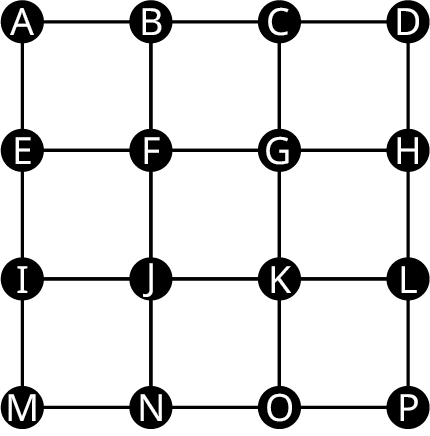1.
ABFGKJFB
2.
GKONJKL
3.
FJKGBA
4.
IJKLKJN
5.
MNOKLH
6.
AFKP
7.
NJFBCGFE
8.
EFJIE
For the following exercises, identify each sequence of vertices from Figure 12.134 as a closed walk, circuit (closed trail), and/or directed cycle (closed path). Select all that apply.
9.
ABFGKJFBA
10.
GKONJKL
11.
FJNOKJIEF
12.
IJKGFEI
13.
MNOKJIM
14.
NJFBCGFJN
15.
ABGFEA
16.
EFGKJFBAE
For the following exercises, use the graphs shown. Identify the graph or graphs with the given characteristics.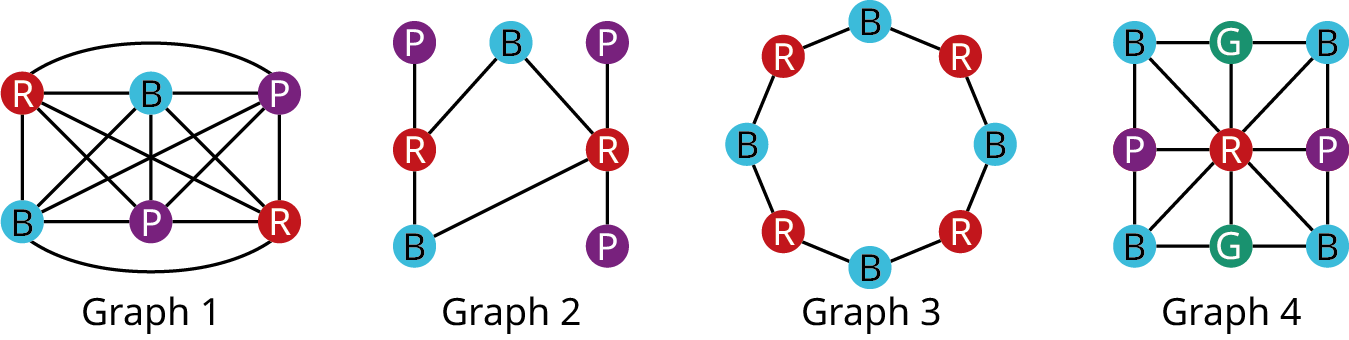17.
The colors do not follow the definition of a graph coloring.
18.
The graph is a two-coloring.
19.
The graph is a three-coloring.
20.
The graph is a four-coloring.
21.
The graph is planar.
22.
The graph has a chromatic number of two.
23.
Fewer colors could be used to color the graph.
24.
The chromatic number is more than four.
25.
The Four-Color Theorem applies to the graph.
For the following exercises, complete the sequence of vertices from the graph to create the indicated kind of closed walk.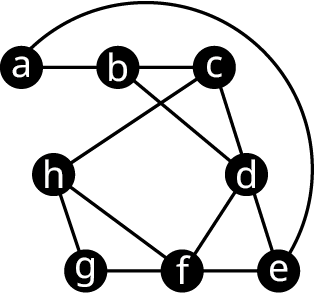26.
Closed path: ab → □ → □ → □ → a
27.
Closed path that visits edge ed twice: ed → □ → □ → □ → e
28.
Directed cycle: ea → □ → □ → □ → e
29.
Directed cycle: bd → □ → □ → □ → b
30.
Circuit that visits vertex d twice: bcd → □ → □ → □ → b
31.
Circuit that visits vertex f twice: ghf → □ → □ → □ → g
For the following exercises, use the graphs shown.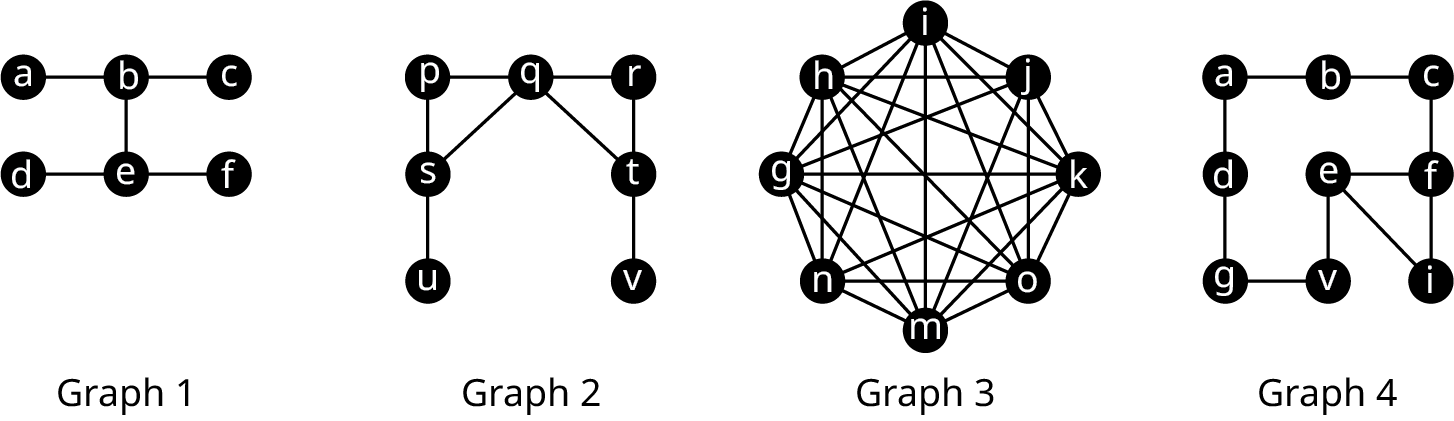32.
Find the chromatic number n for graph 1 and give an n-coloring.
33.
Find the chromatic number n for graph 2 and give an n-coloring.
34.
Find the chromatic number n for graph 3 and give an n-coloring.
35.
Find the chromatic number n for graph 4 and give an n-coloring.
For the following exercises, indicate the smallest and largest possible chromatic number of the graph described.
36.
The graph has 15 vertices and contains a clique with 9 vertices.
37.
A planar graph with 100 vertices and a clique with 3 vertices.
38.
A planar graph with more than 2 vertices and no cliques.
39.
A complete graph with 2,123 vertices.
40.
In chess, a knight can move in any direction, but it must move two spaces then turn and move one more space. The 8 possible moves a knight can make from a space in the center of a five-by-five grid are shown in the first figure. A knight’s tour is a sequence of moves by a knight on a chessboard (of any size) such that the knight visits every square exactly once. If the knight’s tour brings the knight back to its starting position on the board, it is called a closed knight’s tour. Otherwise, it is called an open knight’s tour. Determine if the closed knight’s tour in the second figure is most accurately described as a closed walk, a circuit, or a directed cycle. Explain your reasoning.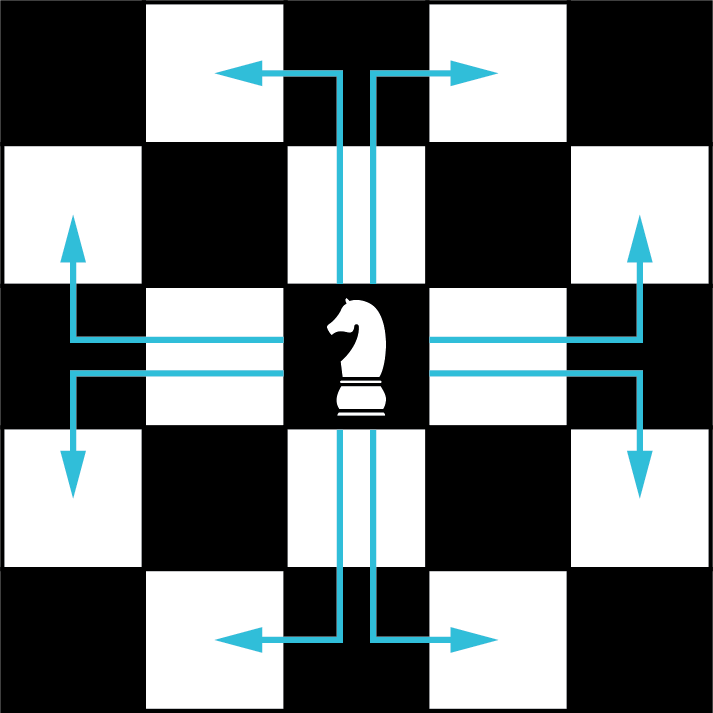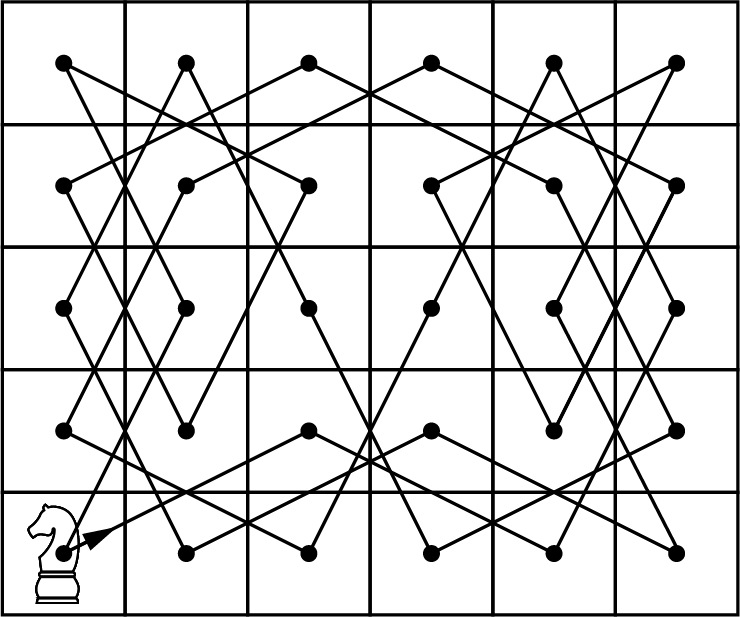41.
An open knight’s tour on a five-by-five board is shown in the figure. Is it most accurately described as a walk, a trail, or a path?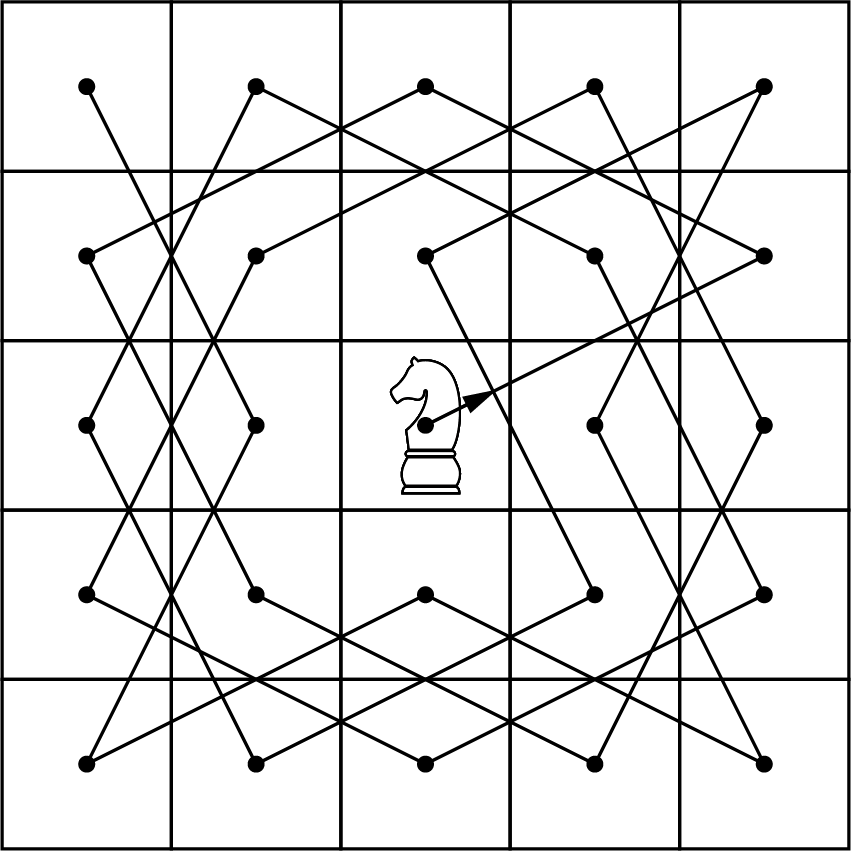42.
A knight’s tour is not possible on a four-by-four board like the one shown in the figure. Find an open tour of the board in which the knight is permitted to travel through a given space more than once to achieve the goal of visiting every space. Is your tour most accurately described as a walk, trail, or path? Explain why.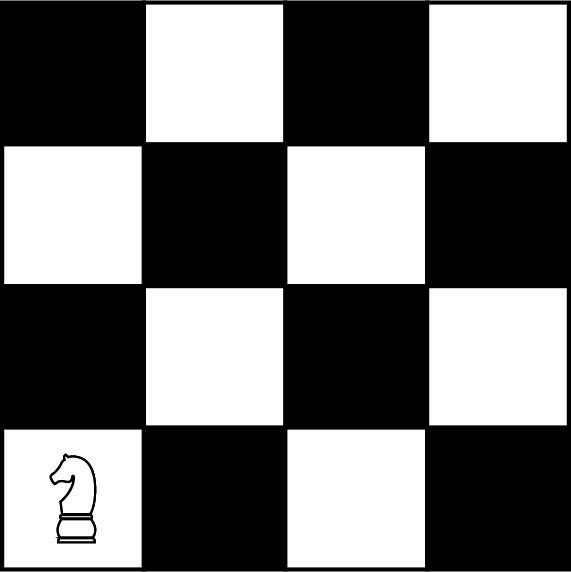The neighborhood of Pines West has three cul-de-sacs that meet at an intersection as shown in the figure. A postal delivery person starts at the intersection and visits each house in a cul-de-sac once, returns to the intersection, visits each house in the next cul-de-sac, and so on, returning to the intersection when finished.
43.
Describe how the route can be represented as a graph. If there is no backtracking, in other words, the person never reverses direction, is the route followed by the postal delivery person best described as a walk, trail, path, closed walk, circuit, or directed cycle? Explain your reasoning.44.
Draw the graph for the route described in the neighborhood. Find its chromatic number. Find a map coloring that shows the chromatic number.
45.
When radio towers are within range of each other, they must use different frequencies. In the graph, the vertices represent the towsers, and the edges indicate the towers are in range of each other. Use graph colorings to determine the number of frequencies needed to avoid two towers in the same range being assigned the same frequency. Give an example of a coloring to support your conclusion.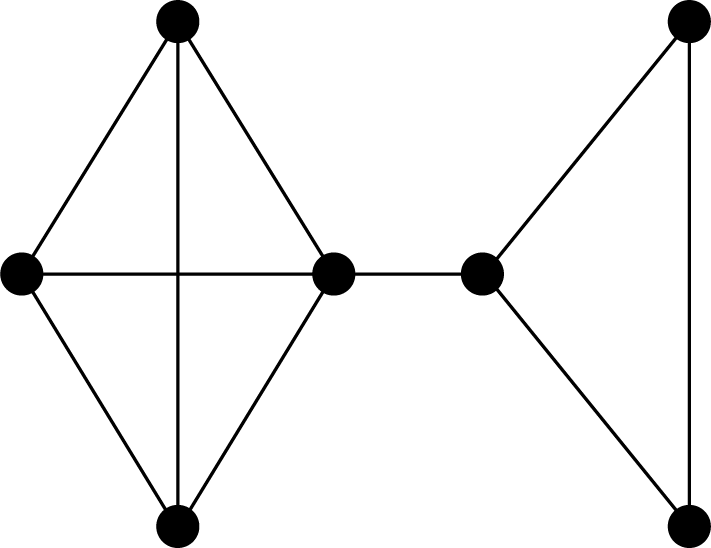46.
A Sudoku puzzle consists of a nine-by-nine grid which is subdivided into nine three-by-three smaller grids. Given an incomplete grid, the goal is to complete it in such a way that each row contains the numbers 1 through 9, each column contains the numbers 1 through 9, and each three by three grid contains the numbers 1 through 9 as shown in the figure. If a graph were drawn with a vertex to represent each entry in the grid, what should the edges represent so that a nine-coloring of the graph would be a solution to the puzzle?At a wedding reception, the bride and groom would like their family and friends to get to know each other better. They have decided that if two people know each other, they will not seat them at the same table. The following is a list of guests and who they know. Guests A, B, C, and D all know each other; guests E, F, G, and H all know each other; guests I, J, and K all know each other; guests P, Q and R all know each other, guests L, M, and O all know each other. Additionally, I knows D and I knows G, J knows M, K knows P, N knows L, and N knows O.
47.
Draw a graph to show the relationships.
48.
Determine the minimum number of tables needed to seat the guests so that they will sit with people they don’t know.
49.
Give a coloring to support your conclusion.
The map of the states of Imaginaria is given. Use the figure to answer the following exercises.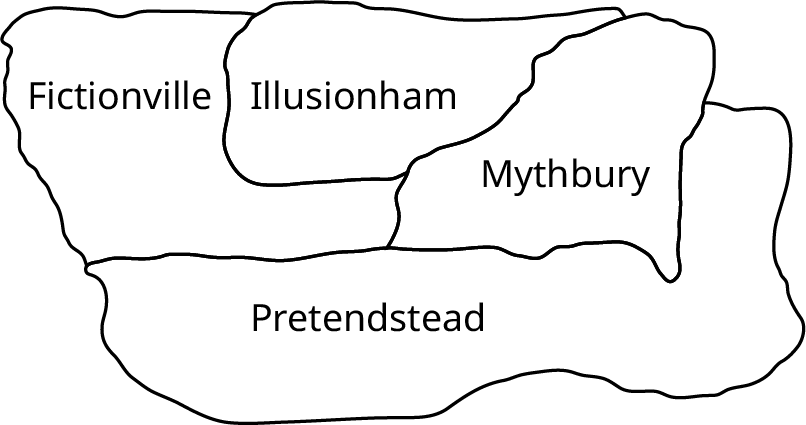50.
Draw a graph to represent the map.
51.
Determine the chromatic number of the graph you found.
52.
Give a graph coloring that supports your answer in the previous question.
53.
Color the provided map to correspond with the coloring you found in the previous question.